# Flowchart

A flowchart is a type of diagram that represents a workflow or process. In research we often want to explain how we recruited our patients, how many that were available from the start, how many that were excluded and how many were left at the final analysis. The Gmisc package provides a convenient set of functions for doing this using the R’s built-in grid package with some bells and whistles. Below is a simple example that illustrates what we’re aiming for.

library(Gmisc, quietly = TRUE)
library(glue)
library(htmlTable)
library(grid)
library(magrittr)

org_cohort <- boxGrob(glue("Stockholm population",
"n = {pop}",
pop = txtInt(1632798),
.sep = "\n"))
eligible <- boxGrob(glue("Eligible",
"n = {pop}",
pop = txtInt(10032),
.sep = "\n"))
included <- boxGrob(glue("Randomized",
"n = {incl}",
incl = txtInt(122),
.sep = "\n"))
grp_a <- boxGrob(glue("Treatment A",
"n = {recr}",
recr = txtInt(43),
.sep = "\n"))

grp_b <- boxGrob(glue("Treatment B",
"n = {recr}",
recr = txtInt(122 - 43 - 30),
.sep = "\n"))

excluded <- boxGrob(glue("Excluded (n = {tot}):",
" - not interested: {uninterested}",
" - contra-indicated: {contra}",
tot = 30,
uninterested = 12,
contra = 30 - 12,
.sep = "\n"),
just = "left")

grid.newpage()
vert <- spreadVertical(org_cohort,
eligible = eligible,
included = included,
grps = grp_a)
grps <- alignVertical(reference = vert$grps, grp_a, grp_b) %>% spreadHorizontal() vert$grps <- NULL

excluded <- moveBox(excluded,
x = .8,
y = coords(vert$included)$top + distance(vert$eligible, vert$included, half = TRUE, center = FALSE))

for (i in 1:(length(vert) - 1)) {
connectGrob(vert[[i]], vert[[i + 1]], type = "vert") %>%
print
}
connectGrob(vert$included, grps[], type = "N") connectGrob(vert$included, grps[], type = "N")

connectGrob(verteligible, excluded, type = "L") # Print boxes vert grps excluded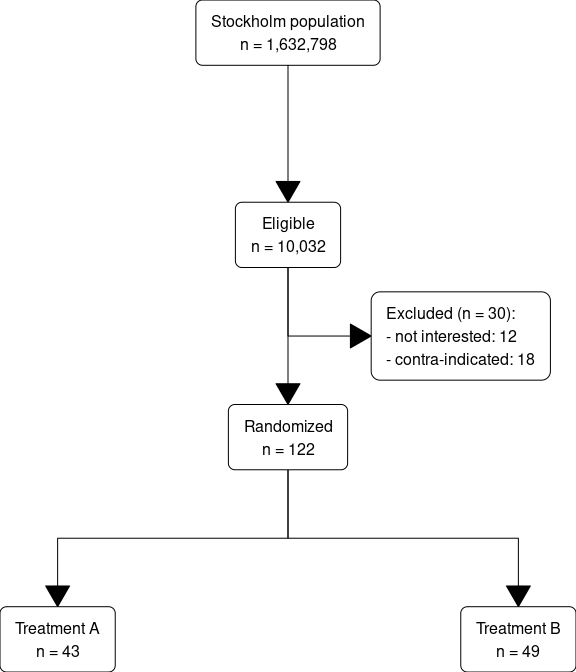# Basic components explained There is a basic set of components that are used for generating flowcharts: • Boxes, these are generated through the boxGrob and boxPropGrob functions. • Arrows between boxes, these are generated through the connectGrob function. These can be positioned directly or preferably manipulated according to the following principles: • Spread - we want to use the full plot and either we position each element or we automatically spread them in a vertical or horizontal direction, spreadHorizontal and spreadVertical functions. • Alignment of boxes - before or after spreading we may want to align boxes: alignHorizontal and alignVertical functions. # A basic box We can start with outputting a single box: grid.newpage() txt <- "Just a plain box with some text - Note that newline is OK" boxGrob(txt)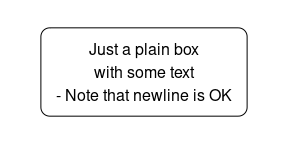We can position and style this box as any element: grid.newpage() boxGrob("A large\noffset\nyellow\nbox", width = .8, height = .8, x = 0, y = 0, bjust = c("left", "bottom"), txt_gp = gpar(col = "darkblue", cex = 2), box_gp = gpar(fill = "lightyellow", col = "darkblue"))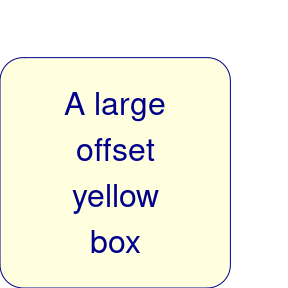# A box with proportiions The boxPropGrob is for displaying proportions as the name indicates. grid.newpage() boxPropGrob("A box with proportions", "Left side", "Right side", prop = .7)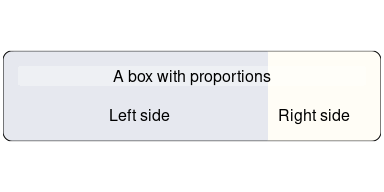# The box coordinates The boxes have coordinates that allow you to easily draw lines to and from it. The coordinates are stored in the coords attribute. Below is an illustration of the coordinates for the two boxes: grid.newpage() smpl_bx <- boxGrob( label = "A simple box", x = .5, y = .9, just = "center") prop_bx <- boxPropGrob( label = "A split box", label_left = "Left side", label_right = "Right side", x = .5, y = .3, prop = .3, just = "center") plot(smpl_bx) plot(prop_bx) smpl_bx_coords <- coords(smpl_bx) grid.circle(y = smpl_bx_coordsy,
x = smpl_bx_coords$x, r = unit(2, "mm"), gp = gpar(fill = "#FFFFFF99", col = "black")) grid.circle(y = smpl_bx_coords$bottom,
x = smpl_bx_coords$right, r = unit(1, "mm"), gp = gpar(fill = "red")) grid.circle(y = smpl_bx_coords$top,
x = smpl_bx_coords$right, r = unit(1, "mm"), gp = gpar(fill = "purple")) grid.circle(y = smpl_bx_coords$bottom,
x = smpl_bx_coords$left, r = unit(1, "mm"), gp = gpar(fill = "blue")) grid.circle(y = smpl_bx_coords$top,
x = smpl_bx_coords$left, r = unit(1, "mm"), gp = gpar(fill = "orange")) prop_bx_coords <- coords(prop_bx) grid.circle(y = prop_bx_coords$y,
x = prop_bx_coords$x, r = unit(2, "mm"), gp = gpar(fill = "#FFFFFF99", col = "black")) grid.circle(y = prop_bx_coords$bottom,
x = prop_bx_coords$right_x, r = unit(1, "mm"), gp = gpar(fill = "red")) grid.circle(y = prop_bx_coords$top,
x = prop_bx_coords$right_x, r = unit(1, "mm"), gp = gpar(fill = "purple")) grid.circle(y = prop_bx_coords$bottom,
x = prop_bx_coords$left_x, r = unit(1, "mm"), gp = gpar(fill = "blue")) grid.circle(y = prop_bx_coords$top,
x = prop_bx_coords$left_x, r = unit(1, "mm"), gp = gpar(fill = "orange")) grid.circle(y = prop_bx_coords$bottom,
x = prop_bx_coords$right, r = unit(2, "mm"), gp = gpar(fill = "red")) grid.circle(y = prop_bx_coords$top,
x = prop_bx_coords$right, r = unit(2, "mm"), gp = gpar(fill = "purple")) grid.circle(y = prop_bx_coords$bottom,
x = prop_bx_coords$left, r = unit(2, "mm"), gp = gpar(fill = "blue")) grid.circle(y = prop_bx_coords$top,
x = prop_bx_coords$left, r = unit(2, "mm"), gp = gpar(fill = "orange"))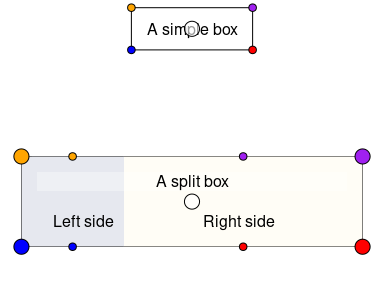# Connecting the boxes In order to make connecting boxes with an arrow there is the connectGrob function. Here’s an example of how you can use it for connecting a set of boxes: grid.newpage() # Initiate the boxes that we want to connect side <- boxPropGrob("Side", "Left", "Right", prop = .3, x = 0, y = .9, bjust = c(0,1)) start <- boxGrob("Top", x = .6, y = coords(side)$y,
box_gp = gpar(fill = "yellow"))
bottom <- boxGrob("Bottom",
x = .6, y = 0,
bjust = "bottom")

sub_side_left <- boxGrob("Left",
x = coords(side)$left_x, y = 0, bjust = "bottom") sub_side_right <- boxGrob("Right", x = coords(side)$right_x,
y = 0,
bjust = "bottom")

odd <- boxGrob("Odd\nbox",
x = coords(side)$right, y = .5) odd2 <- boxGrob("Also odd", x = coords(odd)$right +
distance(bottom, odd, type = "h", half = TRUE) -
unit(2, "mm"),
y = 0,
bjust = c(1,0))

exclude <- boxGrob("Exclude:\n - Too sick\n - Prev. surgery",
x = 1,
y = coords(bottom)top + distance(start, bottom, type = "v", half = TRUE), just = "left", bjust = "right") # Connect the boxes and print/plot them connectGrob(start, bottom, "vertical") connectGrob(start, side, "horizontal") connectGrob(bottom, odd, "Z", "l") connectGrob(odd, odd2, "N", "l") connectGrob(side, sub_side_left, "v", "l") connectGrob(side, sub_side_right, "v", "r") connectGrob(start, exclude, "-", lty_gp = gpar(lwd = 2, col = "darkred", fill = "darkred")) # Print the grobs start bottom side exclude sub_side_left sub_side_right odd odd2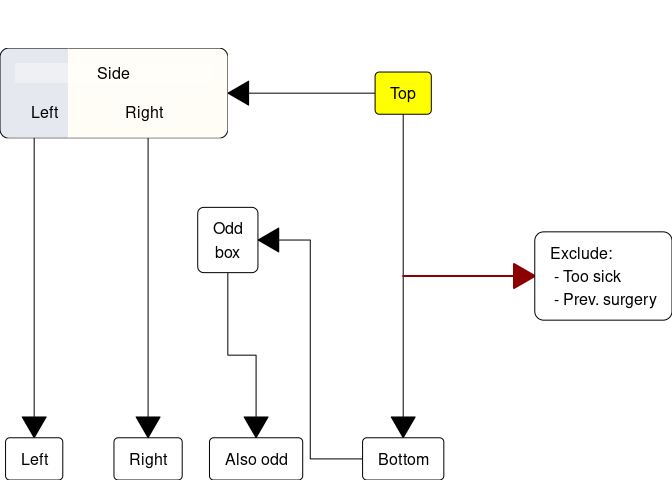# Alignment We frequently want to align boxes in either a horizontal or a vertical row. For this there are two functions, alignHorizontal() and alignVertical(). align_1 <- boxGrob("Align 1", y = .9, x = 0, bjust = c(0), box_gp = gpar(fill = "#E6E8EF")) align_2 <- boxPropGrob("Align 2", "Placebo", "Treatment", prop = .7, y = .8, x = .5) align_3 <- boxGrob("Align 3\nvertical\ntext", y = 1, x = 1, bjust = c(1, 1), box_gp = gpar(fill = "#E6E8EF")) b1 <- boxGrob("B1", y = .3, x = .1, bjust = c(0)) b2 <- boxGrob("B2 with long\ndescription", y = .6, x = .5) b3 <- boxGrob("B3", y = .2, x = .8, bjust = c(0, 1)) grid.newpage() align_1 alignHorizontal(reference = align_1, b1, b2, b3, .position = "left") align_2 alignHorizontal(reference = align_2, b1, b2, b3, .position = "center", .sub_position = "left") alignHorizontal(reference = align_2, b1, b2, b3, .position = "left", .sub_position = "right") align_3 alignHorizontal(reference = align_3, b1, b2, b3, .position = "right")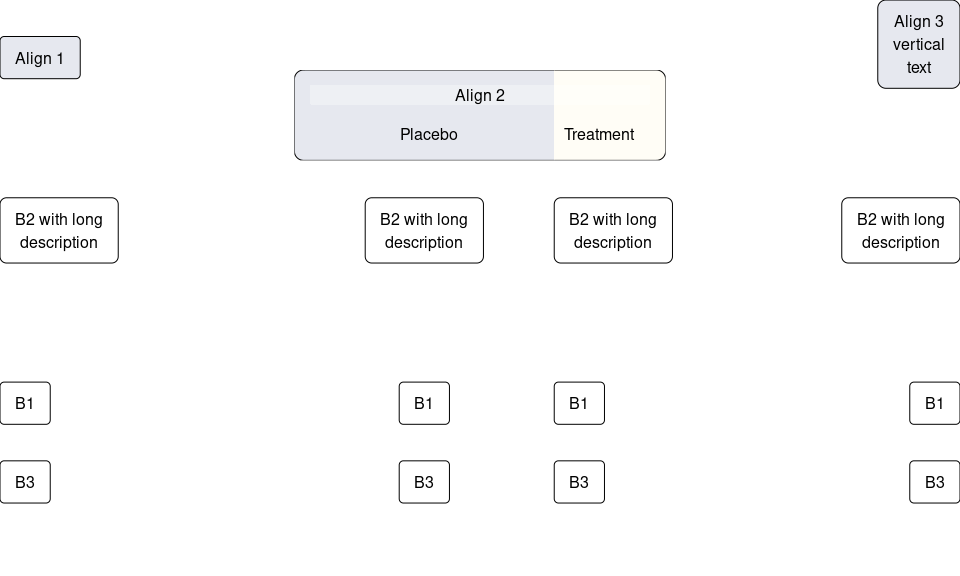Here are similar examples of vertical alignment: align_1 <- boxGrob("Align 1\nvertical\ntext", y = 1, x = 1, bjust = c(1, 1), box_gp = gpar(fill = "#E6E8EF")) align_2 <- boxPropGrob("Align 2", "Placebo", "Treatment", prop = .7, y = .5, x = .6) align_3 <- boxGrob("Align 3", y = 0, x = 0, bjust = c(0, 0), box_gp = gpar(fill = "#E6E8EF")) b1 <- boxGrob("B1", y = .3, x = 0.1, bjust = c(0, 0)) b2 <- boxGrob("B2 with long\ndescription", y = .6, x = .3) b3 <- boxGrob("B3", y = .2, x = .85, bjust = c(0, 1)) grid.newpage() align_1 alignVertical(reference = align_1, b1, b2, b3, .position = "top") align_2 alignVertical(reference = align_2, b1, b2, b3, .position = "center") align_3 alignVertical(reference = align_3, b1, b2, b3, .position = "bottom")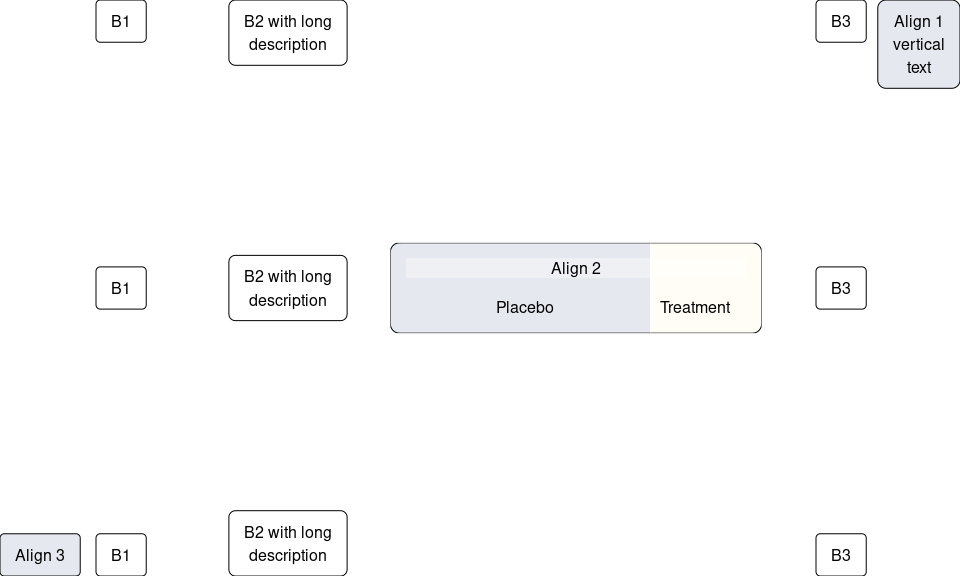# Spreading Similarly to alignment we often want to spread our boxes within a space so that we use all the available space in the viewport. This can be done through the spreadHorizontal() and spreadVertical(). You can both spread the entire span or only between a subspan that is defined using the .to and .from arguments. b1 <- boxGrob("B1", y = .85, x = 0.1, bjust = c(0, 0)) b2 <- boxGrob("B2", y = .65, x = .6) b3 <- boxGrob("B3", y = .45, x = .6) b4 <- boxGrob("B4 with long\ndescription", y = .7, x = .8) from <- boxGrob("from", y = .25, x = .05, box_gp = gpar(fill = "darkgreen"), txt_gp = gpar(col = "white")) to <- boxGrob("to this wide box", y = coords(from)y,
x = .95,
bjust = "right",
box_gp = gpar(fill = "darkred"),
txt_gp = gpar(col = "white"))

txtOut <- function(txt, refBx) {
grid.text(txt,
x = unit(2, "mm"),
y = coords(refBx)$top + unit(2, "mm"), just = c("left", "bottom")) grid.lines(y = coords(refBx)$top + unit(1, "mm"),
gp = gpar(col = "grey"))
}
grid.newpage()
txtOut("Basic", b1)
alignVertical(reference = b1,
b1, b2, b3, b4,
.position = "top") %>%
spreadHorizontal()

txtOut("From-to", b2)
alignVertical(reference = b2,
b1, b2, b3, b4,
.position = "top") %>%
spreadHorizontal(.from = .2,
.to = .7)

txtOut("From-to with center and reverse the box order", b3)
alignVertical(reference = b3,
b1, b2, b3, b4,
.position = "top") %>%
spreadHorizontal(.from = .7,
.to = .2,
.type = "center")

txtOut("Between boxes", from)
from
to
alignVertical(reference = from,
b1, b2, b3, b4,
.position = "top") %>%
spreadHorizontal(.from = from,
.to = to)

# Now we switch the order and set the type to center the distance between the boxes
bottom_from <- moveBox(from, x = coords(to)$right, y = 0, just = c(1, 0)) bottom_to <- moveBox(to, x = coords(from)$left, y = 0, just = c(0, 0))
bottom_from
bottom_to
alignVertical(reference = bottom_from,
b1, b2, b3, b4,
.position = "bottom") %>%
spreadHorizontal(.from = bottom_from,
.to = bottom_to,
.type = "center")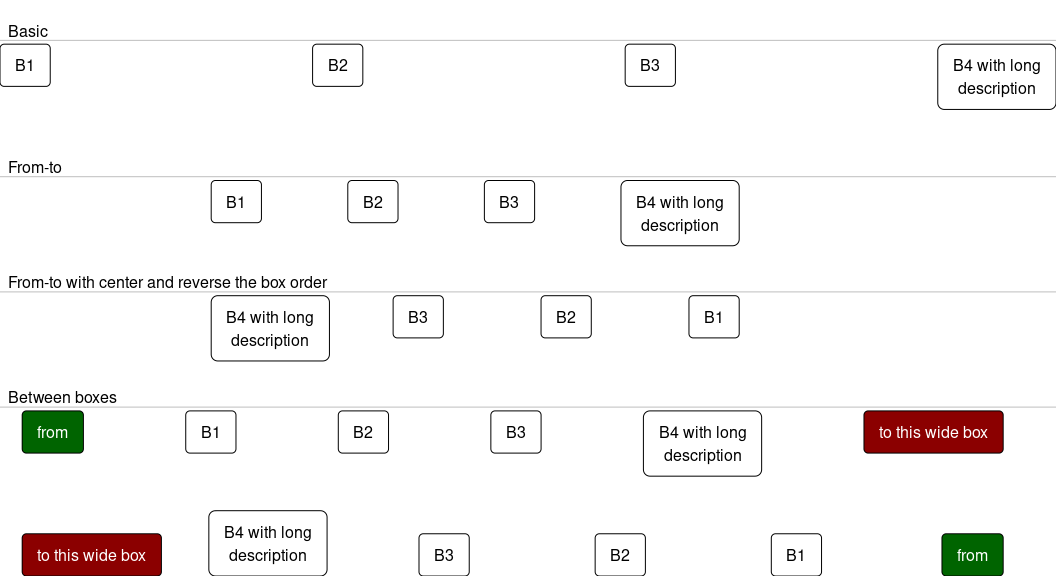Vertical spreading follows the same pattern:

b1 <- boxGrob("B1",
y = .8,
x = 0.1,
bjust = c(0, 0))
b2 <- boxGrob("B2 with long\ndescription",
y = .5,
x = .5)
b3 <- boxGrob("B3",
y = .2,
x = .8)
b4 <- boxGrob("B4",
y = .7,
x = .8)

txtOut <- function(txt, refBx) {
grid.text(txt,
x = coords(refBx)$left - unit(2, "mm"), y = .5, just = c("center", "bottom"), rot = 90) grid.lines(x = coords(refBx)$left - unit(1, "mm"),
gp = gpar(col = "grey"))
}

grid.newpage()
txtOut("Basic", b1)
alignHorizontal(reference = b1,
b1, b2, b3, b4,
.position = "left") %>%
spreadVertical()

txtOut("From-to", b2)
alignHorizontal(reference = b2,
b1, b2, b3, b4,
.position = "left") %>%
spreadVertical(.from = .2,
.to = .7)

txtOut("From-to with center and reverse the box order", b3)
alignHorizontal(reference = b3,
b1, b2, b3, b4,
.position = "left") %>%
spreadVertical(.from = .7,
.to = .2,
.type = "center")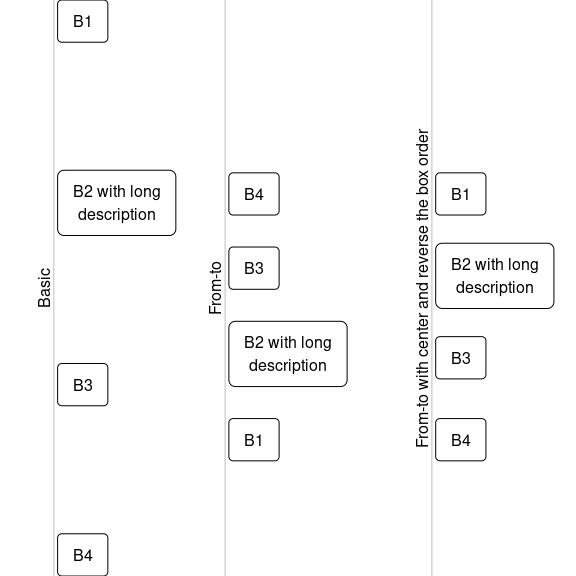# Math expressions in boxes

It is possible to use the R expression or the bquote functions to produce bold or italics text, or even formulas.

A few pointers on expression

• expressions with multiple elements should be combined using paste. E.g. expression(paste(beta, "1")) would produce $$\beta1$$
• the behavior of paste when used in expression is more like the normal behavior or paste0 (i.e. no separating space)
• Greek letters can be entered outside of quotes by typing the name e.g. expression(beta) will become $$\beta$$ and expression(Gamma) will become $$\Gamma$$ (note the case, not all Greek letters are available in upper case)
• superscripts are done via expression(x^2) and subscripts via expression(x)
grid.newpage()
###############
# Expressions #
###############
# Font style
alignVertical(
reference = 1,
.position = "top",
boxGrob(expression(bold("Bold text"))),
boxGrob(expression(italic("Italics text"))),
boxGrob(expression(paste("Mixed: ", italic("Italics"), " and ", bold("bold"))))) %>%
spreadHorizontal

# Math
alignVertical(
reference = .5,
boxGrob(expression(paste("y = ", beta, " + ", beta, X, " + ", beta, X^2))),
boxGrob(expression(sum(n, i == 1, x) %subset% " \u211D")),
boxGrob(expression(beta ~~ gamma ~~ Gamma))) %>%
spreadHorizontal

##########
# Quotes #
##########
a = 5
alignVertical(
reference = 0,
.position = "bottom",
bquote(alpha == theta * .(a) + ldots) %>% boxGrob,
paste("argument", sQuote("x"), "\nmust be non-zero") %>% boxGrob) %>%
spreadHorizontal(.from = .2, .to = .8)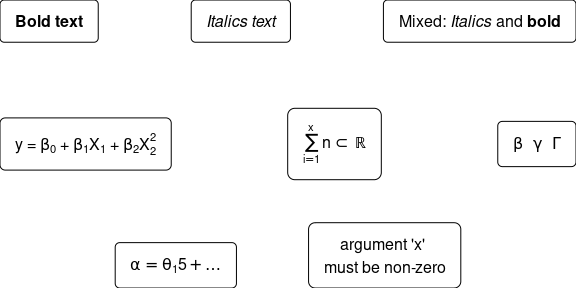See the plotmath help file for more details.

# Grid & some background info

The grid package is what makes R graphics great. All the popular tools with awesome graphics use the grid as the back-end, e.g. ggplot2 and lattice. When I started working on the forestplot package I first encountered the grid and it was instant love. In this vignette I’ll show how you can use the flowchart-functions in this package together with grid in order to generate a flowchart.

## Basics

The grid package splits the plot into views. You can define a viewport and it will work as an isolated part of the plot, ignorant of the world around it. You do this via viewport(), below I create a plot and add a rectangle to it:

# Load the grid library
# part of standard R libraries so no need installing
library(grid)

# Create a new graph
grid.newpage()

pushViewport(viewport(width = .5, height = .8))

grid.rect(gp = gpar(fill = "#D8F0D1"))

popViewport()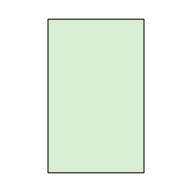Important to note is that the grid allows you to define precise units or relative units.

## Relative units

• npc - ranges from 0-1 where 1 is 100% of the viewport width.
• snpc - similar to npc but is the same length in height/width.
• lines - the height of a line. The go-to method if you want to know the height of a few lines of text. It’s relative to the viewport’s fontsize and lineheight.
• char - the lines without the lineheight part.

Below we draw a line with relative units in two nested viewports. Note that the to lines are generated from the exact same grob object but appear different depending on the viewport they are in:

grid.newpage()
pushViewport(viewport(width = .5, height = .8, clip = "on"))
grid.rect(gp = gpar(lty = 2, fill = "lightyellow"))
lg <- linesGrob(x = unit(c(.2, 1), "npc"),
y = unit(c(.2, 1), "npc"),
gp = gpar(lwd = 2))
grid.draw(lg)
pushViewport(viewport(x = 0, y = .6, just = "left", width = .4, height = .4, angle = 20))
grid.rect(gp = gpar(fill = "lightblue")) # A translucent box to indicate the new viewport
grid.draw(lg)
popViewport()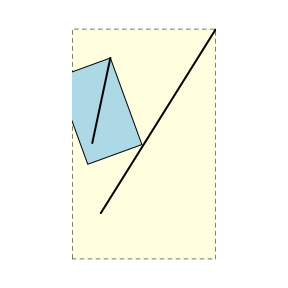## Absolute units

• mm - probably my go-to unit when I want something absolute.
• inch - if you prefer inches I guess this is the go-to choice.

Below we draw a line with absolute units in two nested viewports. Note that the lines have the exact same length:

grid.newpage()
pushViewport(viewport(width = .5, height = .8, clip = "on"))
grid.rect(gp = gpar(lty = 2, fill = "lightyellow"))
lg <- linesGrob(x = unit(c(2, 10), "mm"),
y = unit(c(2, 10), "mm"),
gp = gpar(lwd = 2))
grid.draw(lg)
pushViewport(viewport(x = 0, y = .6, just = "left", width = .4, height = .4, angle = 20))
grid.rect(gp = gpar(fill = "lightblue")) # A translucent box to indicate the new viewport
grid.draw(lg)
popViewport()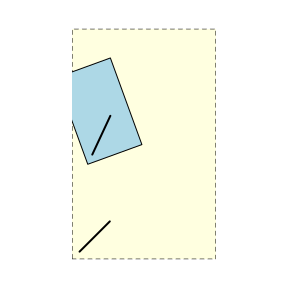# Tips for debugging

If you find that your elements don’t look as expected make sure that your not changing viewport/device. While most coordinates are relative some of them need to be fixed and therefore changing the viewport may impact where elements are rendered.